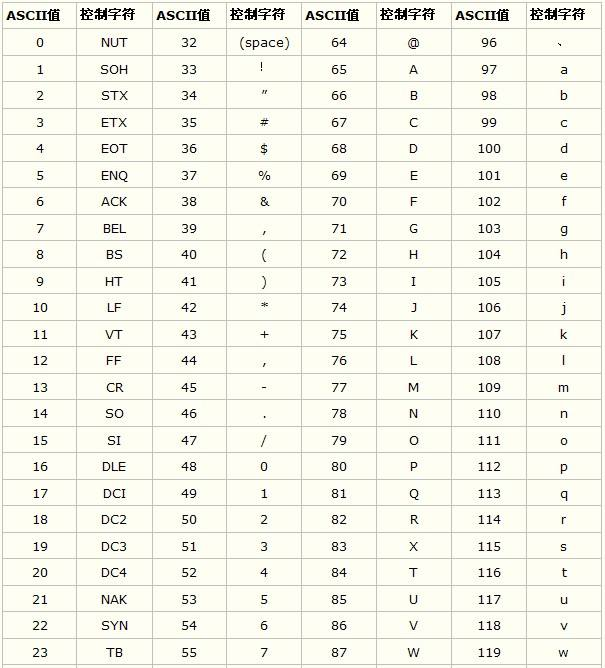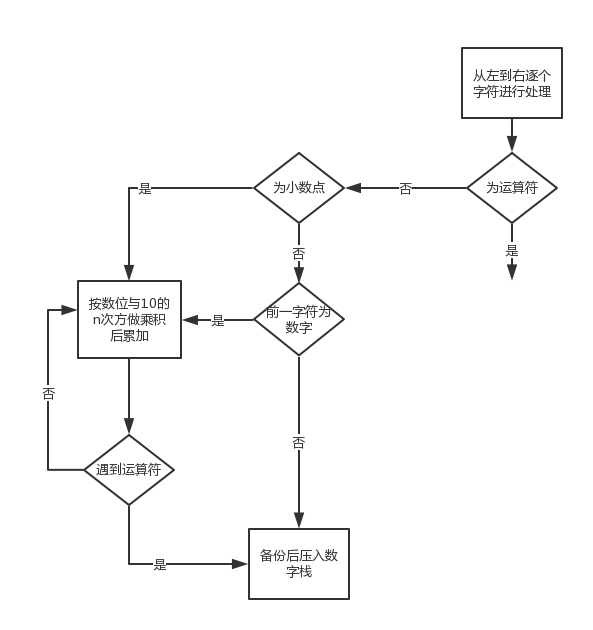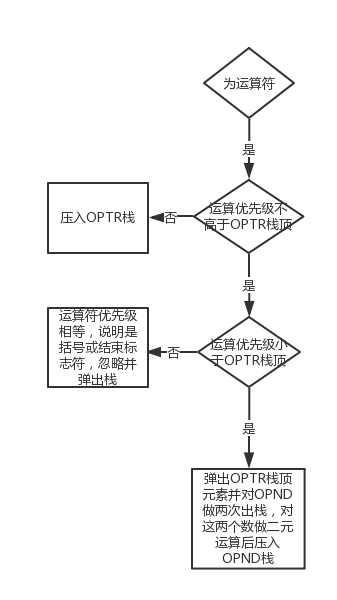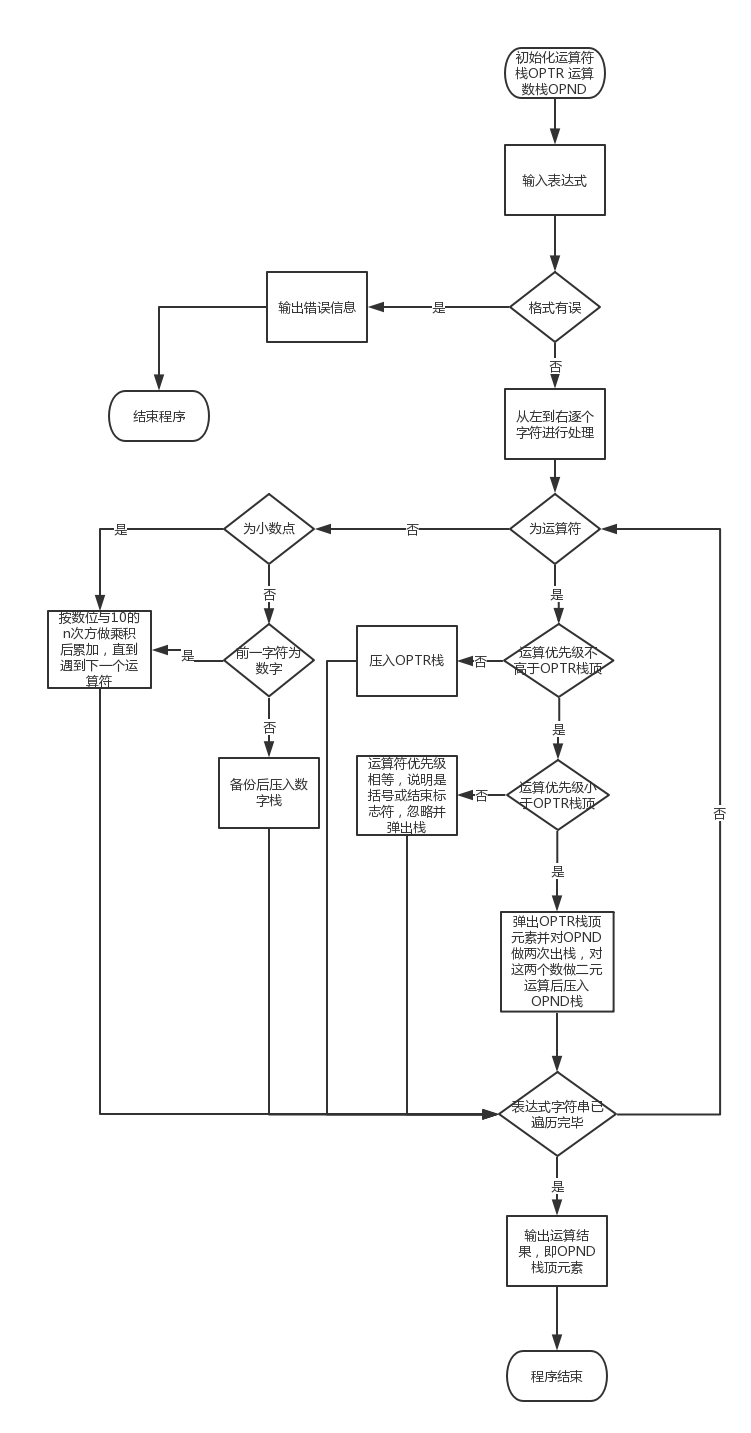## （C语言实现）栈求表达式的值（实数范围内）_栈求表达式的值ns图_清水三千的博客-程序员秘密

• 初始化
• 出栈
• 入栈
• 取栈顶
• 判空

1.数字或小数点（非运算符）2.运算符

``````char Precede(char c1,char c2){
//判定运算符的栈顶运算符与读入的运算符之间优先关系
char c;
switch(c1){
case '+':
case '-':
switch(c2){
case '+':
case '-':
case ')':
case '#':
c='>';
break;
default:
c='<';
}
break;
case '*':
case '/':
if(c2=='('){
c='<';
}
else{
c='>';
}
break;
case '(':
if(c2==')'){
c='=';
}
else{
c='<';
}
break;
case ')':
c='>';
break;
case '#':
if(c2=='#'){
c='=';
}
else{
c='<';
}
}
return c;
}
````````````//[email protected] 12/18
#include <stdio.h>
#include <stdlib.h>
#include <math.h>
#include <string.h>
#define MAXSIZE 100
//double栈，用于存放运算数
typedef struct {
double data[MAXSIZE];
int top;
}Stack_n;
//char栈，用于存放运算符
typedef struct {
char data[MAXSIZE];
int top;
}Stack_c;
//栈的初始化
void Init_n(Stack_n s);
void Init_c(Stack_c s);

int IsEmpty_n(Stack_n s);
int IsEmpty_c(Stack_c s);
//入栈函数
void Push_n(Stack_n *s,double e);
void Push_c(Stack_c *s,char e);
//出栈函数
void Pop_n(Stack_n *s,double *e);
void Pop_c(Stack_c *s,char *e);
//取栈顶函数
double GetTop_n(Stack_n s);
char GetTop_c(Stack_c s);

char Precede(char c1,char c2);//判断优先级
double Operate(double a,char theta,double b);//二元运算
int In(char c,char *OP);//是否为 + - * / ( )
void check(char *s);//用于检查输入的多项式格式是否正确
double Poland(char * s);//主要功能实现函数

char OP={'+','-','*','/','(',')','#'}; //运算符数组(因多个函数使用故声明为公有的)

int main(){
char s;
printf("请输入表达式：\n");
gets(s);
double r = Poland(s);
printf("%1.2lf\n",r);
return 0;
}

void Init_n(Stack_n *s){
s->top = 0;
}

void Init_c(Stack_c *s){
s->top = 0;
}
//栈的判空函数
int IsEmpty_n(Stack_n s){
if(s.top == 0){
return 1;
}
else{
return 0;
}
}
int IsEmpty_c(Stack_c s){
if(s.top == 0){
return 1;
}
else{
return 0;
}
}
//入栈函数
void Push_n(Stack_n *s,double e){
s->top++;
s->data[s->top] = e;
}
void Push_c(Stack_c *s,char e){
s->top++;
s->data[s->top] = e;
}
//出栈函数
void Pop_n(Stack_n *s,double *e){
*e = s->data[s->top];
s->top--;
}
void Pop_c(Stack_c *s,char *e){
*e = s->data[s->top];
s->top--;
}
//取栈顶函数
double GetTop_n(Stack_n s){
return s.data[s.top];
}
char GetTop_c(Stack_c s){
return s.data[s.top];
}

char Precede(char c1,char c2){
//判定运算符的栈顶运算符与读入的运算符之间优先关系
char c;
switch(c1){
case '+':
case '-':
switch(c2){
case '+':
case '-':
case ')':
case '#':
c='>';
break;
default:
c='<';
}
break;
case '*':
case '/':
if(c2=='('){
c='<';
}
else{
c='>';
}
break;
case '(':
if(c2==')'){
c='=';
}
else{
c='<';
}
break;
case ')':
c='>';
break;
case '#':
if(c2=='#'){
c='=';
}
else{
c='<';
}
}
return c;
}

double Operate(double a,char theta,double b){
//进行二元运算 a theta b
double sum;
switch(theta)
{
case '+':
sum=a+b;
break;
case '-':
sum=a-b;
break;
case '*':
sum=a*b;
break;
case '/':
sum=a/b;
break;
}
return sum;
}

int In(char c,char *OP){
//判断是不是运算符
for(int i=0;i<7;i++)
if(c==OP[i])   //是运算符
return 1;
return 0;
}
//判断思路：当前字符是运算符且前一位或后一位也是运算符且这3位都不为‘（’和‘）’时说明连续输入了两个运算符
void check(char *s){
char *t = s;
for(int i = 0;i < strlen(s);i++){
if(i > 0 && i < strlen(s) - 1 && In(s[i],OP) && (In(s[i - 1],OP) || In(s[i + 1],OP))
&&(s[i] != '(' && s[i] != ')') && (s[i - 1] != '(' && s[i - 1] != ')')
&&(s[i + 1] != '(' && s[i + 1] != ')')){
printf("输入格式有误！！请检查是否存在连续输入了运算符等错误！！\n");
getchar();
exit(0);
}
}
}

double Poland(char * s){
//对多项式格式进行检查
check(s);
//进行多项式的求值以及后缀表达式的输出
int i=0,len,flag=0;
double a,b,sum;
char c1=s,e;

Stack_c OPTR; 	//运算符栈
Stack_n OPND; 	//运算数栈
Init_c(&OPTR);  //初始化栈
Init_n(&OPND);

len=strlen(s);
s[len]='#';
s[len+1]='\0';
Push_c(&OPTR,'#');//补‘#’作为结束标志
while(s[i]!='#'||GetTop_c(OPTR)!='#'){ //遍历每一个字符 为‘#’结束循环
if(s[i] == ' '){
i++;
continue;
}
if(!In(s[i],OP)){ //如果不是运算符
if(c1=='.'){//如果上一个字符是小数点
flag++;
}
if(flag){
double t;
Pop_n(&OPND,&t);
Push_n(&OPND,t + (double)(s[i] - '0') / pow(10,flag));//对小数点后的部分 按位数运算并入栈
flag++;
}
if(s[i]!='.'&&!flag){
if(!In(c1,OP)&&!IsEmpty_n(OPND)){//如果上一个字符是数字（说明是多位数） 根据位数运算后入栈
double t;
Pop_n(&OPND,&t);
Push_n(&OPND,t * 10 + s[i] - '0');
}
else//否则压入运算数栈
Push_n(&OPND,(double)(s[i]-'0'));
}
c1=s[i++]; //读取下一个字符
}
else{//如果是运算符
flag=0;
switch(Precede(GetTop_c(OPTR),s[i])){
case '>':  //当前运算符优先级低
Pop_c(&OPTR,&e); //运算符出栈,和操作数的头两个数运算
Pop_n(&OPND,&b);
Pop_n(&OPND,&a);
Push_n(&OPND,Operate(a,e,b)); //计算结果入栈
break;
case '=': //优先级相等
Pop_c(&OPTR,&e); //弹出运算符栈顶元素
c1 = s[i++];
break;
case '<':
Push_c(&OPTR,s[i]); //当前运算符优先级高 入栈
c1 = s[i++];
break;
}
}
}
sum=GetTop_n(OPND);
return sum; //返回运算数栈顶元素，即运算结果
}//@@ end @@``````### Chrome浏览器命令行启动参数_chrome 启动参数 打开网页_liuyukuan的博客-程序员秘密

Chrome浏览器命令行启动参数http://blog.csdn.net/qq_32786873/article/details/70173265http://blog.csdn.net/u012593626/article/details/44540485 1 2 说明 如何使用这些参数&lt;br&gt;chrome 谷歌浏览器命令行大全...